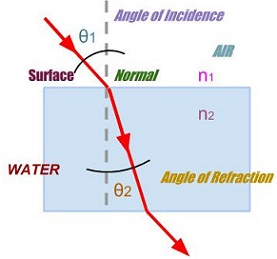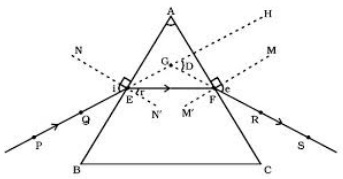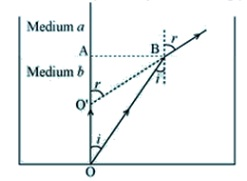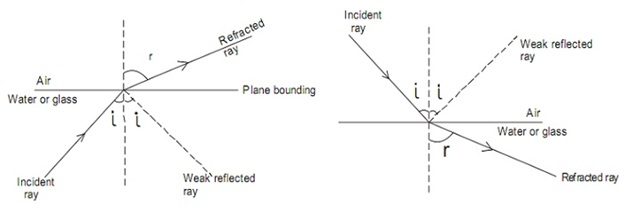#### Refraction of Light, Physics tutorial

Refraction of Light:

Refraction is basically the bending of waves as they pass from one medium to the other, due to a change in their speed. The phenomenon is most generally related with light, however can as well apply to sound, or even water, waves. It occurs if a series of waves travels toward the new medium at an angle, in such a way that one side undergoes a change in speed before the other, causing it to turn toward the slower side in the similar manner that a moving vehicle will tend to turn when one side is slowed more than the other. The Refraction can cause objects to appear displaced, and might amplify distant sounds. It consists of numerous uses in the context of light, like as prisms and lenses.

Refractive Index:

Each and every medium via which waves can move consists of a refractive index which points out how fast they will travel. In case of light, this is found by dividing its speed in a vacuum through its speed in that specific medium. This is a ratio between the speeds of the mediums; therefore it is not measured in any unit. The refractive index usually rises by the density of the medium: it is one for a vacuum and is more than one for all identified natural materials.

Uses:

The most general uses of refraction are in prisms and lenses. A lens is designed in such a way that light entering it is focused through refraction toward a point, generating a magnified image of an object. Lenses can be employed in telescopes or binoculars to attained detailed images of distant objects, or in magnifying glasses and microscopes to observe extremely small objects, like microorganisms not visible to the naked eye. A prism can be employed to split light up into various colors in much similar way as water droplets make a rainbow, however giving a more precise image which can be employed to examine the light source in detail.

Refraction of Light through Rectangular Glass Block:If light travels from one medium to the other, it usually bends or refracts. The law of refraction gives us a manner of predicting the amount of bend. The law is more complex than that for reflection; however an understanding of refraction will be essential for our future discussion of lenses and their applications. The law of refraction is as well termed as Snell's law, named for Willobrord Snell, who proposed the law in the year 1621.

Snell's law introduces the relationship between angles of incidence and refraction for a wave imposing on an interface between two media having different index of refraction. The law obeys from the boundary condition which a wave is continuous across a boundary that needs that the stage of the wave be constant on any particular plane, resultant in:

Snell's law:

n1sinθ1 = n2sinθ2

Or, μ = n2/n1 = sinθ1/sinθ2

Here μ is a constant termed as refractive index.

Rule 1: If a light ray travels from a rarer medium to the denser medium, the light ray bends in the direction of the normal.

Rule 2: If a light ray travels from a denser medium to a rarer medium, the light ray bends away from the normal.

Refraction of Light through Triangular Prism:PE - Incident ray                   ∠i - Angle of incidence

EF - Refracted ray                 ∠r - Angle of refraction

FS - Emergent ray                 ∠e - Angle of emergence

∠A - Angle of the prism         ∠D - Angle of deviation

Let, consider a triangular glass prism. It consists of two triangular bases and three rectangular lateral surfaces. Such surfaces are inclined to one other. The angle between its two lateral faces is termed as angle of prism.

In this, PE is the incident ray, EF is the refracted ray and FS is the emergent ray. You might note that a ray of light is entering from air to glass at first surface AB. The light ray on refraction has bent in the direction of normal. At the second surface AC, the light ray has entered from glass to the air. Therefore it has bent away from the normal. The peculiar shape of the prism forms the emergent ray bend at an angle to the direction of incident ray. This angle is termed as the angle of deviation. In this case D is the angle of deviation.

The angle of deviation mainly based on:

• The angle of incidence
• The refracting angle (A) of the prism
• The refractive index of the material of the prism

Laws of Refraction:

Law 1: The incident ray, the refracted ray and the normal to the surface at the point of incidence all lie in one plane.

Law 2: For any two specific pair of media, the ratio of the sine of the angle of incidence to the sine of the angle of refraction is a constant. The law is as well termed as Snell's law after the scientist Willebrod Snellius who first proposed it.

n1 sin θ1 = n2 sin θ2

Here,

θ1 is the angle of incidence

θ2 is the angle of refraction

n1 is the absolute index of refraction of the incident medium

n2 is the absolute index of refraction of the refractive medium

Real and Apparent Depths:

Real depth: It is the actual or real distance of an object below the surface, as would be computed by submerging a perfect ruler all along with it.

Apparent depth: If a person looks at a straight object, like a pencil or straw that is placed at a slant, partly in water, the object appears to bend at the surface of water. This is due to the bending of light rays as they move from the water to air. Once the rays arrive at the eye, the eye traces them back as straight lines (that is, lines of sight). The lines of sight intersect at a higher position than where the real rays originated. This reasons the pencil to appear higher and the water to appear shallower than it actually is. The depth which the water appears to be if viewed from above is termed as the apparent depth.

Relation between Real and Apparent Depths:The refractive index of a medium 'a' with respect to medium 'b' is given by:

bμa = sin i/sin r => aμb = sin r/sin i

In ΔOAB, sin i = AB/OB

In ΔO'AB, sin r = AB/O'B

aμb = (AB/O'B)/(AB/OB) = (AB/O'B) x (OB/AB) = OB/O'B

As the point A and B are very close, OB ≈ OA (real depth) and O'B ≈ O'A (apparent depth)

aμb = Real depth/Apparent depth

Measurement of Refractive Index:

1) By snell's law:

n1 sin θ1 = n2 sin θ2

Here,

θ1 is the angle of incidence

θ2 is the angle of refraction

n1 is the absolute index of refraction of the incident medium

n2 is the absolute index of refraction of the refractive medium

2) By Real and apparent depths:

Refraction index = real thickness (depth)/apparent thickness (depth)

Critical Angle and Total Internal Reflection:

Whenever light is incident on the interface between two media, there is generally a weak reflected ray in addition to the refracted ray.A striking change takes place if the situation is reversed optically in such a way that light passes from glass to air.

If light passes via an optical dense medium to meet up the surface of less dense one, as the angle of incidence 'i' is increased, the angle of refraction 'r' as well increases until it is 90o. In that case, the refracted ray is all along the interface (surface) of the two media.

=> Critical angle 'c' is the angle of incidence in the denser medium for which the angle of refraction in less dense medium is 90o

=> The value of critical angle 'c' based on the nature of two media or their relative refractive indices. (It is around 42o for crown glass the most general kind of optical glass).

=> Total internal reflection takes place if the incident angle 'i' is more than the critical angle 'c'. At this point all incidents light is reflected back to provide strong reflected ray. There is no refraction.

Total internal reflection can't take place if light is incident in air on a glass block. This is due to the reason that the angle of refraction in the glass is for all time less than the angle of incidence. Thus, even when the angle of incidence in air is almost 90o, a refracted ray is achieved.

Reflection always takes place at air-glass boundary however this reflection is just partial because most of the light passes into the glass (or water). It is thus significant to note that: For total internal reflection to take place,

a) Light should travel from an optically denser medium to the optically less dense medium.

b) The angle of incidence in denser medium should be greater than that of the critical angle.

Relationship between Critical angle C and Total Internal Reflection:

Let's state that the less dense medium is air (n=1). Then the refractive index of the second medium is:

Refractive index = sin i/sin r

Refractive index = sin 90°/sin c

Refractive index = 1/sin c

Here, c is the critical angle for the medium

Applications of total Internal Reflection:

1) Total internal reflection is the operating principle of the optical fibers that are utilized in the endoscopes and telecommunications.

2) It is the operating principle of automotive rain sensors that control automatic windscreen or windshield wipers.

3) The other application of total internal reflection is the spatial filtering of light.

4) Prisms in binoculars utilize total internal reflection, instead of reflective coatings, to fold optical paths and exhibit erect images.

4) Several multi-touch screens utilize frustrated total internal reflection in combination by a camera and suitable software to pick up multiple targets.

5) Gonioscopy utilizes total internal reflection to view the anatomical angle formed between the cornea of eye and iris.

Tutorsglobe: A way to secure high grade in your curriculum (Online Tutoring)

Expand your confidence, grow study skills and improve your grades.

Since 2009, Tutorsglobe has proactively helped millions of students to get better grades in school, college or university and score well in competitive tests with live, one-on-one online tutoring.

Using an advanced developed tutoring system providing little or no wait time, the students are connected on-demand with a tutor at www.tutorsglobe.com. Students work one-on-one, in real-time with a tutor, communicating and studying using a virtual whiteboard technology.  Scientific and mathematical notation, symbols, geometric figures, graphing and freehand drawing can be rendered quickly and easily in the advanced whiteboard.

Free to know our price and packages for online physics tutoring. Chat with us or submit request at info@tutorsglobe.com